# Online Algebra 2

## Get 1 on 1 help from Algebra 2 tutors

Algebra 2 is a class that builds upon the coursework and foundations that were laid out in their Pre-Algebra and Algebra 1 classes. It is also the next step in preparing for later mathematics classes, such as Pre-Calculus and Calculus.

When your student is working with Algebra 2 problems and coursework, they will cover a wide range in different topics, from developing an understanding of matrices to solving logarithmic functions. Using concepts taught in earlier math courses, they will apply and build upon them.

In Algebra 2, your student will better understand different types of equations, and they will practice solving for different variables. Your student will also practice with graphing functions, such as learning to interpret an exponential graph. Many school systems combine Algebra 2 with the study of Trigonometry, although even if the courses are not combined, your student is likely to learn some of the basic properties of Trigonometry, such as utilizing equations such as Pythagorean’s Theorem.

### Algebra 2

Algebra 2 is a class that builds upon the coursework and foundations that were laid out in their Pre-Algebra and Algebra 1 classes. It is also the next step in preparing for later mathematics classes, such as Pre-Calculus and Calculus. When your student is working with Algebra 2 problems and coursework, they will cover a wide range in different topics, from developing an understanding of matrices to solving logarithmic functions. Using concepts taught in earlier math courses, they will apply and build upon them.

In Algebra 2, your student will better understand different types of equations, and they will practice solving for different variables. Your student will also practice with graphing functions, such as learning to interpret an exponential graph. Completing this course successfully will help prepare your student for future coursework in mathematics, such as Pre-Calculus. Many school systems combine Algebra 2 with the study of Trigonometry, although even if the courses are not combined, your student is likely to learn some of the basic properties of Trigonometry, such as utilizing equations such as Pythagorean’s Theorem.

### Studying Algebra 2

When your student works with one of our tutors in one-on-one tutoring, your student will get plenty of guidance in Algebra 2 topics. Rather than glossing over topics as is often the case in classrooms filled with students to cover all of the material before an end-of-year test, our tutors will dive deep into the subject material to make sure that your student understands and learns the necessary material before moving on to the next topic. Between studying the different concepts and working with their tutor on practice problems, your student will have the opportunity to learn everything they need to know about Algebra 2 before they move on to future coursework.

A firm understanding of Algebra 2, as well as related topics such as Trigonometry, are needed before your student moves on to more abstract mathematics coursework, such as Calculus. As this course is generally taught in high school, your student may be pulled in numerous different directions by their studies and preparing for college. Our tutors can help your student focus their studies and provide much needed direction when learning the material. Your student will go from learning how to solve basic equations for variables to a more detailed knowledge in topics such as quadratic equations. Our tutors will help them master one area before they move on to another with our one-on-one tutoring environment.

### Learn Algebra 2 With Us

Through our one-on-one tutoring environment, your student is able to get a strong grasp on the increasingly tough coursework students face when they are in Algebra 2. Your student will benefit from in a one-on-one learning environment, where our tutors are able to focus on what your student’s needs are. Rather than being torn in different directions by a classroom full of students as many teachers face, our tutors will work at your student’s pace. Through our provided instruction, your student will get the basics that they need to succeed, and then have a deeper dive into the material as necessary for their coursework. We lay out the information they need and provide guidance from the very start.

Our tutors are able to help your student no matter what the problems are that they face, from basic subject material such as understanding right angles to more complex quadratic equations. Our one-on-one tutoring system allows us to walk your student through each concept and work with them to solve problems. Our tutors can then quiz them on material to ensure a firm grasp on the necessary topics.

Regardless of whether you need an Algebra 2 tutor or a tutor who is experienced in Algebra 2 and Trigonometry, your student will have someone who can help them every step of the way. Troublesome areas can get addressed sooner rather than later, so your student doesn’t find out that they have a problem when they get tested on the material. Our tutors will work with your student to make sure that they learn the material in a way that is most effective for their learning style. For example, more visual learners can be taught with graphs and charts, while an auditory learner and a tutor may do better with a lecture style format to the tutoring sessions.

Our tutoring program is effective no matter what course of study student is it. As your student learns material in the classroom, our tutors can work with them to apply these concepts to problems and examples. If your student has trouble with the given problem, our tutors are able to go back over the material and spend as much time as needed to make sure your student understands the concepts. Contact us today to see how we can help your student with Algebra 2.Same Tutor
Every TimeTutors with
MS DegreeSame
TextbooksManaged
TutoringVery
ConvenientAffordable
\$16/hr for 8hr Monthly
Below 8hr/Month, \$20/hr
What You’ll Learn
• Matrices
• Quadratic equations
• Logarithmic equations
• Inequalities
• Exponential equations•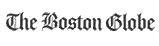•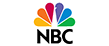••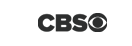•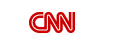•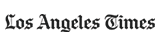•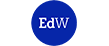•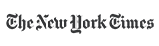•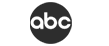### How You Benefit FromThis Program

• Flexible Scheduling
• Quality Learning
• Proven Results
• Tutors with Masters
• Affordable Price
• Dedicated Support
• Money Back Guarantee

### I’m Interested in This Program

By registering here I accept Growing Stars' Terms of Use and Privacy Policy.
Please verify.
Validation complete :)
Validation failed :(